# Twu-Sim-Tassone equation of state

Twu, Sim and Tassone presented a cubic equation of state for accurate representation of hydrocarbons that has become known as the Twu-Sim-Tassone or TST equation of state. With a critical compressibility factor of (Eq. 5)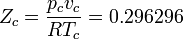$Z_c = \frac{p_cv_c}{RT_c} = 0.296296$

it better represents the compressibility than many of than the Redlich-Kwong equation of states, including the Soave modified version, and the Peng and Robinson equation of state.

The equation follows the general cubic form resulting in the equation (Eq. 2):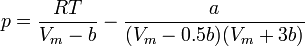$p=\frac{RT}{V_m-b}-\frac{a}{(V_m-0.5b)(V_m+3b)}$

where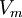$V_m$ is the molar volume,$R$ is the molar gas constant,$T$ is the temperature,$p$ is the pressure, and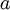$a$ and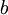$b$ are the attractive and repulsive parameters akin to those of the Van der Waals equation of state. Relations exists between$a$ and$b$ and the critical parameters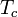$T_c$ and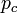$p_c$ in the forms (Eqs. 3 and 4):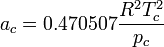$a_c=0.470507 \frac{ R^2T_c^2}{p_c}$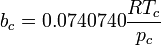$b_c=0.0740740 \frac{ RT_c}{p_c}$